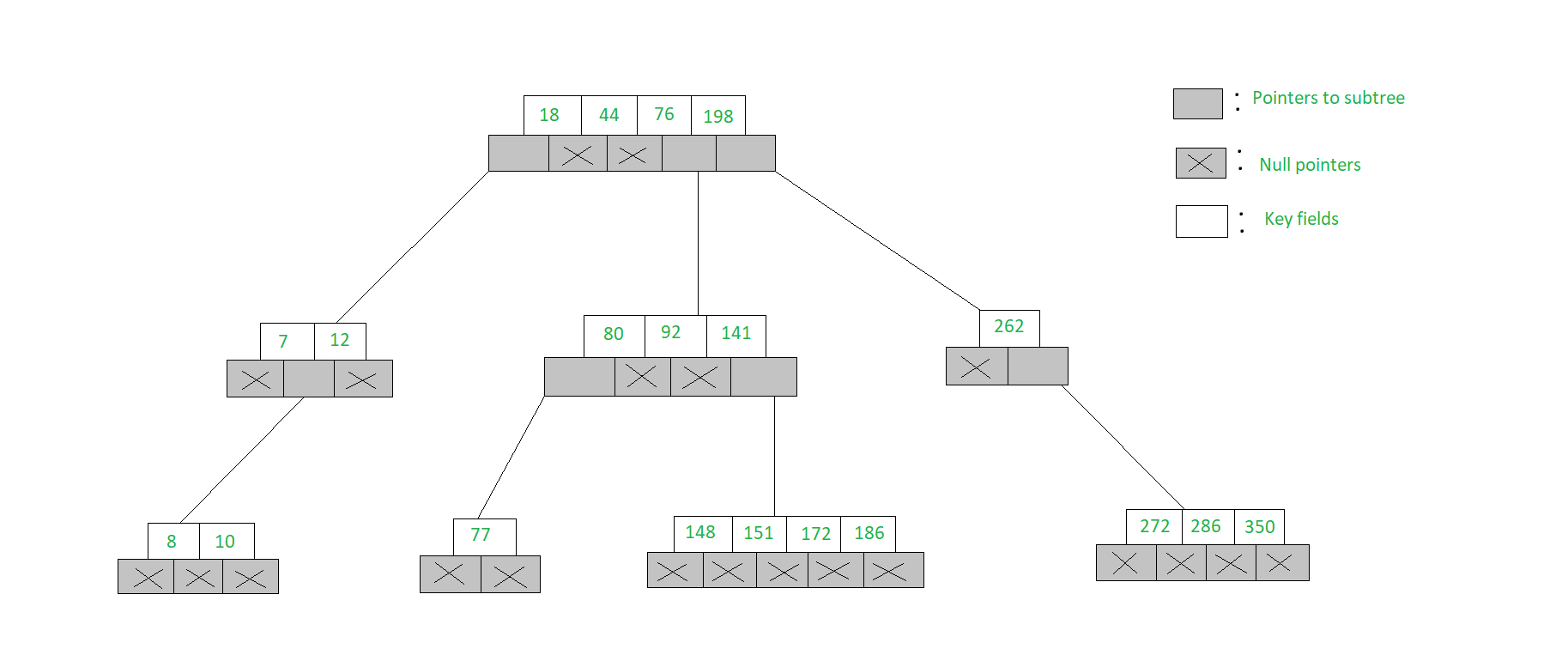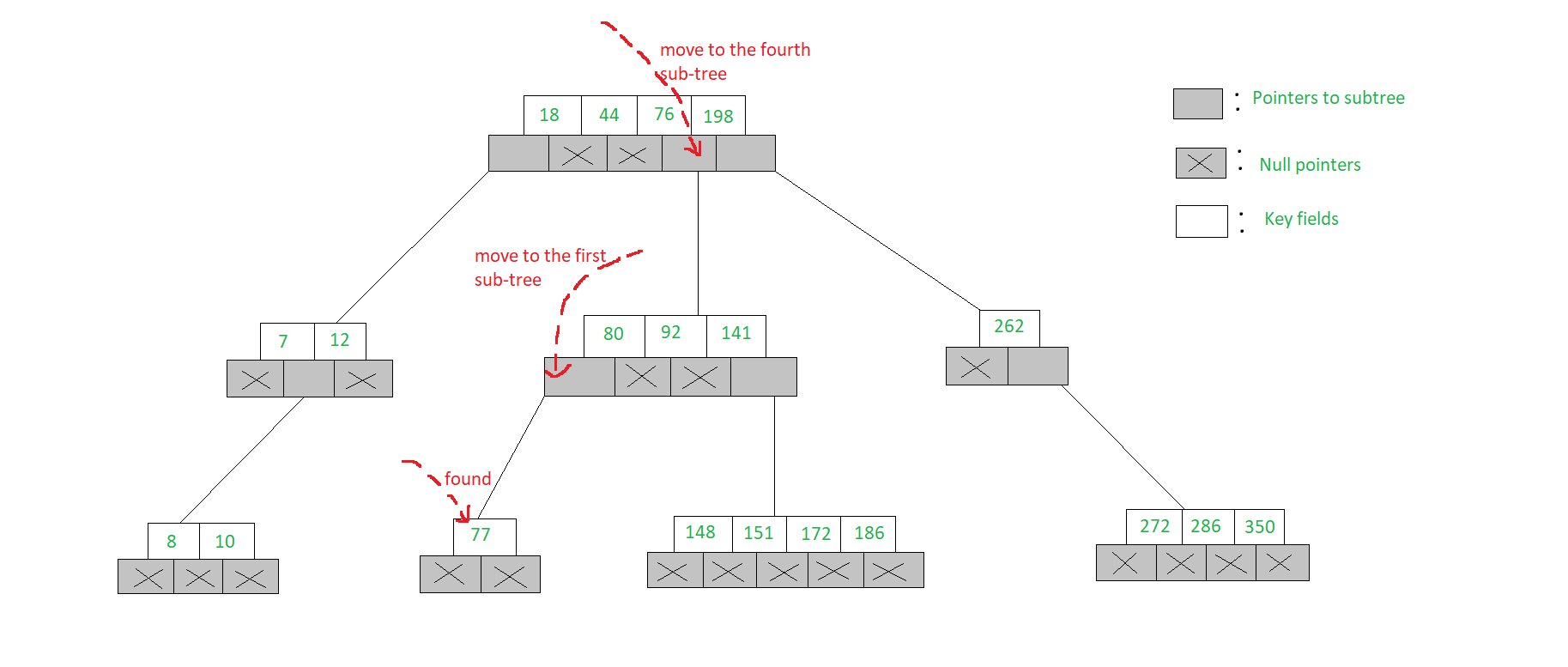# m-WAY Search Trees | Set-1 ( Searching )

The m-way search trees are multi-way trees which are generalised versions of binary trees where each node contains multiple elements. In an m-Way tree of order m, each node contains a maximum of m – 1 elements and m children.

The goal of m-Way search tree of height h calls for O(h) no. of accesses for an insert/delete/retrieval operation. Hence, it ensures that the height h is close to log_m(n + 1).

The number of elements in an m-Way search tree of height h ranges from a minimum of h to a maximum of.

An m-Way search tree of n elements ranges from a minimum height of log_m(n+1) to a maximum of n

An example of a 5-Way search tree is shown in the figure below. Observe how each node has at most 5 child nodes & therefore has at most 4 keys contained in it.The structure of a node of an m-Way tree is given below:

 `struct` `node { ` `    ``int` `count; ` `    ``int` `value[MAX + 1]; ` `    ``struct` `node* child[MAX + 1]; ` `}; `

• Here, count represents the number of children that a particular node has
• The values of a node stored in the array value
• The addresses of child nodes are stored in the child array
• The MAX macro signifies the maximum number of values that a particular node can contain

Searching in an m-Way search tree:

• Searching for a key in an m-Way search tree is similar to that of binary search tree
• To search for 77 in the 5-Way search tree, shown in the figure, we begin at the root & as 77> 76> 44> 18, move to the fourth sub-tree
• In the root node of the fourth sub-tree, 77< 80 & therefore we move to the first sub-tree of the node. Since 77 is available in the only node of this sub-tree, we claim 77 was successfully searched`// Searches value in the node ` `struct` `node* search(``int` `val, ` `                    ``struct` `node* root, ` `                    ``int``* pos) ` `{ ` ` `  `    ``// if root is Null then return ` `    ``if` `(root == NULL) ` `        ``return` `NULL; ` `    ``else` `{ ` ` `  `        ``// if node is found ` `        ``if` `(searchnode(val, root, pos)) ` `            ``return` `root; ` ` `  `        ``// if not then search in child nodes ` `        ``else` `            ``return` `search(val, ` `                          ``root->child[*pos], ` `                          ``pos); ` `    ``} ` `} ` ` `  `// Searches the node ` `int` `searchnode(``int` `val, ` `               ``struct` `node* n, ` `               ``int``* pos) ` `{ ` `    ``// if val is less than node->value ` `    ``if` `(val < n->value) { ` `        ``*pos = 0; ` `        ``return` `0; ` `    ``} ` ` `  `    ``// if the val is greater ` `    ``else` `{ ` `        ``*pos = n->count; ` ` `  `        ``// check in the child array ` `        ``// for correct position ` `        ``while` `((val < n->value[*pos]) ` `               ``&& *pos > 1) ` `            ``(*pos)--; ` `        ``if` `(val == n->value[*pos]) ` `            ``return` `1; ` `        ``else` `            ``return` `0; ` `    ``} ` `} `

search():

• The function search() receives three parameters
• The first parameter is the value to be searched, second is the address of the node from where the search is to be performed and third is the address of a variable that is used to store the position of the value once found
• Initially a condition is checked whether the address of the node being searched is NULL
• If it is, then simply a NULL value is returned
• Otherwise, a function searchnode() is called which actually searches the given value
• If the search is successful the address of the node in which the value is found is returned
• If the search is unsuccessful then a recursive call is made to the search() function for the child of the current node

searchnode():

• The function searchnode() receives three parameters
• The first parameter is the value that is to be searched
• The second parameter is the address of the node in which the search is to be performed and third is a pointer pos that holds the address of a variable in which the position of the value that once found is stored
• This function returns a value 0 if the search is unsuccessful and 1 if it is successful
• In this function initially it is checked whether the value that is to be searched is less than the very first value of the node
• If it is then it indicates that the value is not present in the current node. Hence, a value 0 is assigned in the variable that is pointed to by pos and 0 is returned, as the search is unsuccessful

Don’t stop now and take your learning to the next level. Learn all the important concepts of Data Structures and Algorithms with the help of the most trusted course: DSA Self Paced. Become industry ready at a student-friendly price.

My Personal Notes arrow_drop_upCheck out this Author's contributed articles.

If you like GeeksforGeeks and would like to contribute, you can also write an article using contribute.geeksforgeeks.org or mail your article to contribute@geeksforgeeks.org. See your article appearing on the GeeksforGeeks main page and help other Geeks.

Please Improve this article if you find anything incorrect by clicking on the "Improve Article" button below.